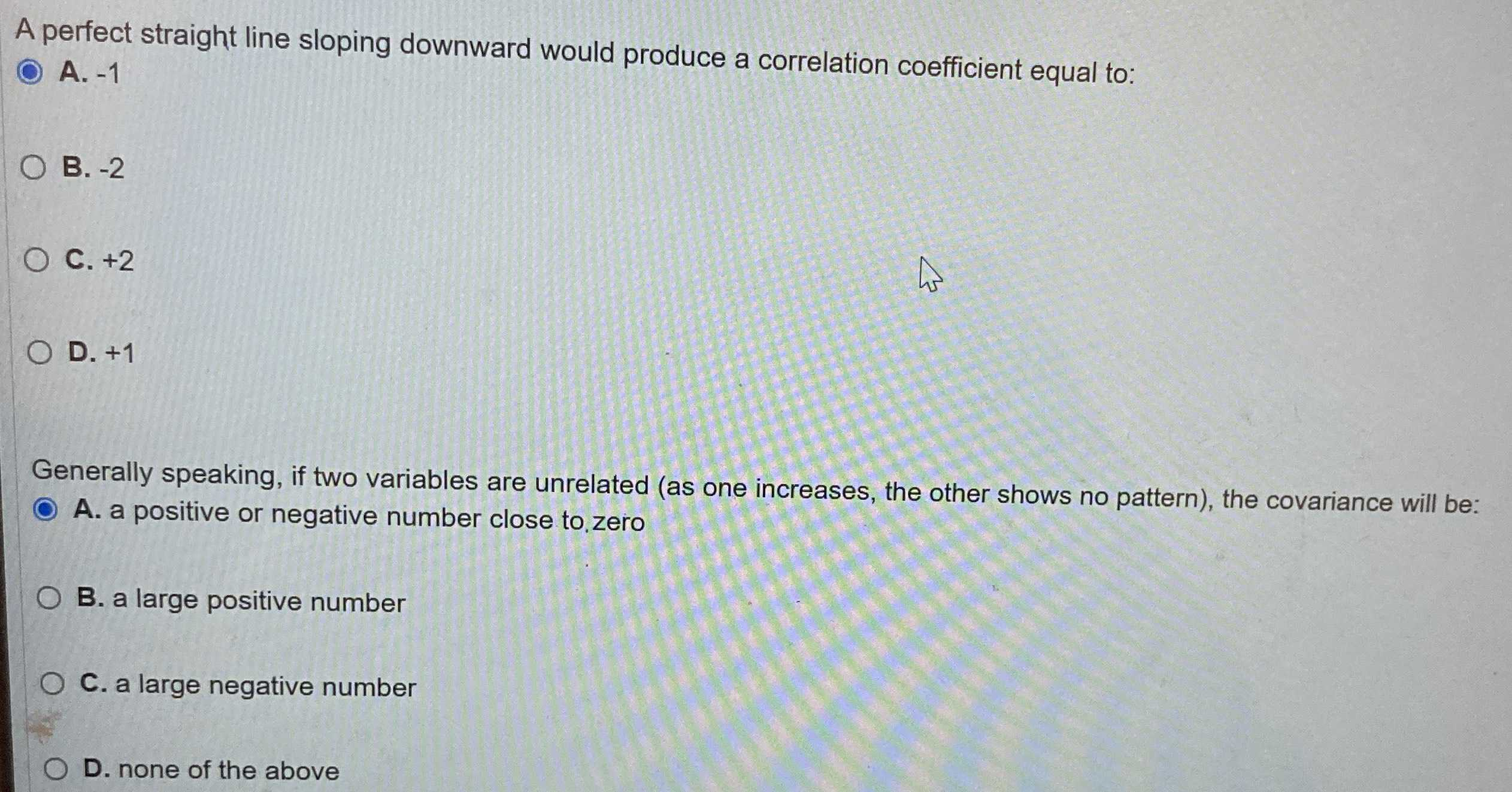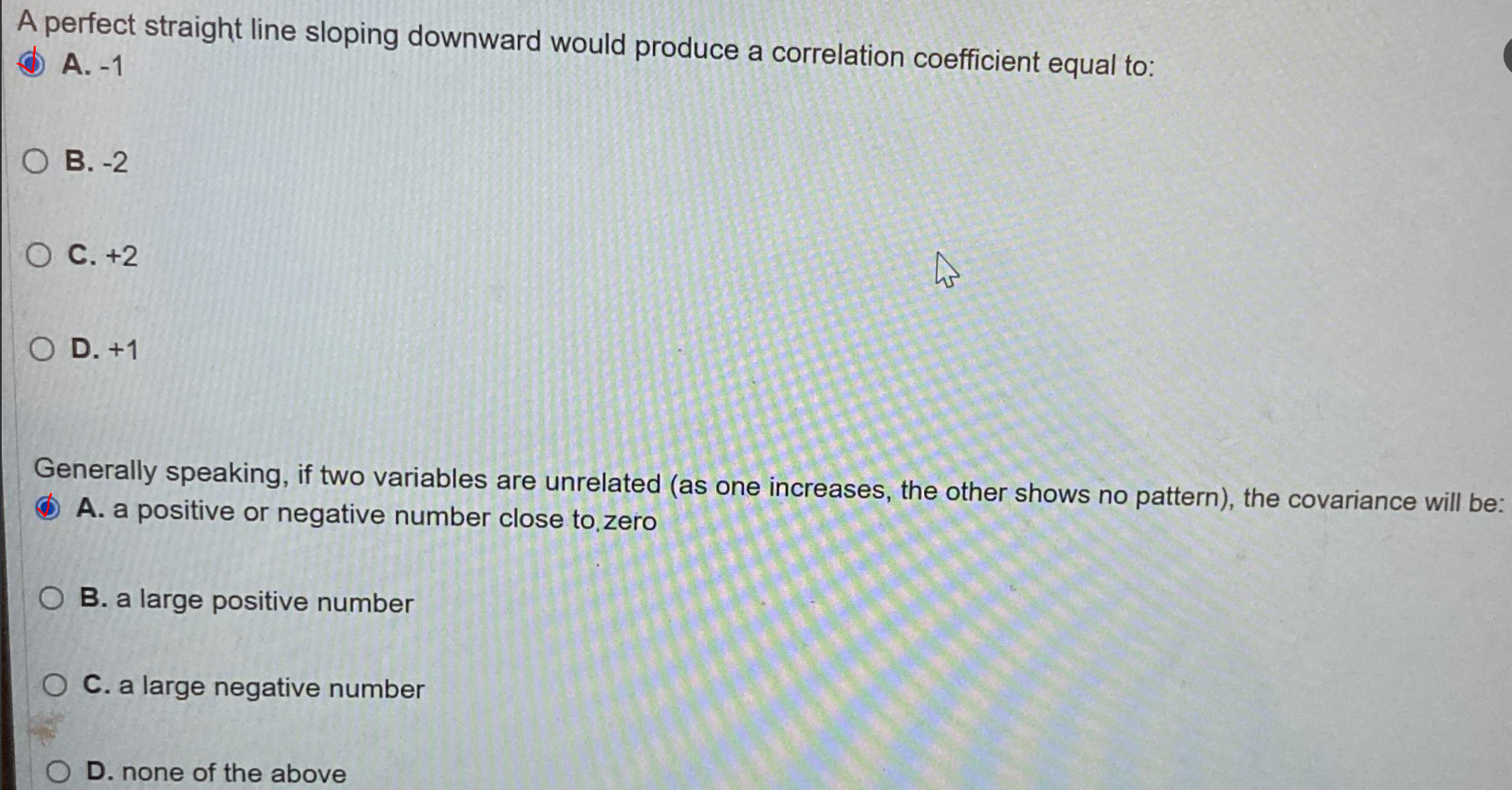### ¿Todavía tienes preguntas de matemáticas?

Pregunte a nuestros tutores expertos
Algebra
PreguntaA perfect straight line sloping downward would produce a correlation coefficient equal to:

A. $$- 1$$

B. $$- 2$$

C. $$+ 2$$

D. $$+ 1$$

Generally speaking, if two variables are unrelated (as one increases, the other shows no pattern), the covariance will be:

A. a positive or negative number close to.zero

B. a large positive number

C. a large negative number

D. none of the above